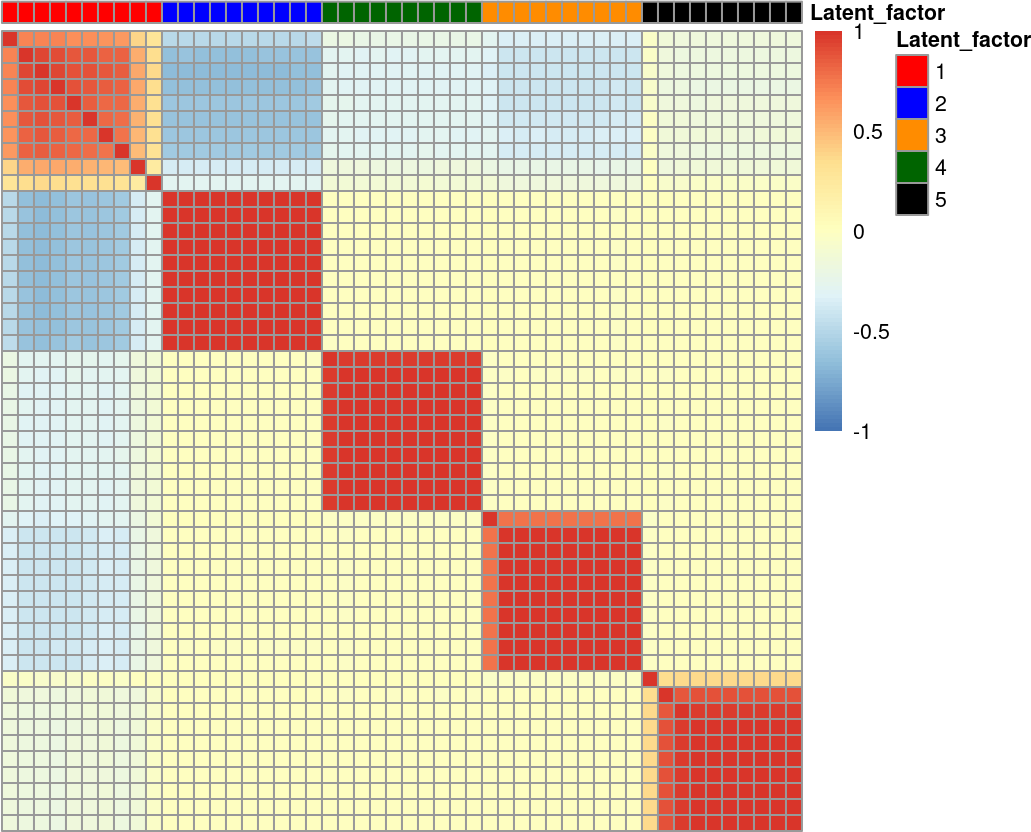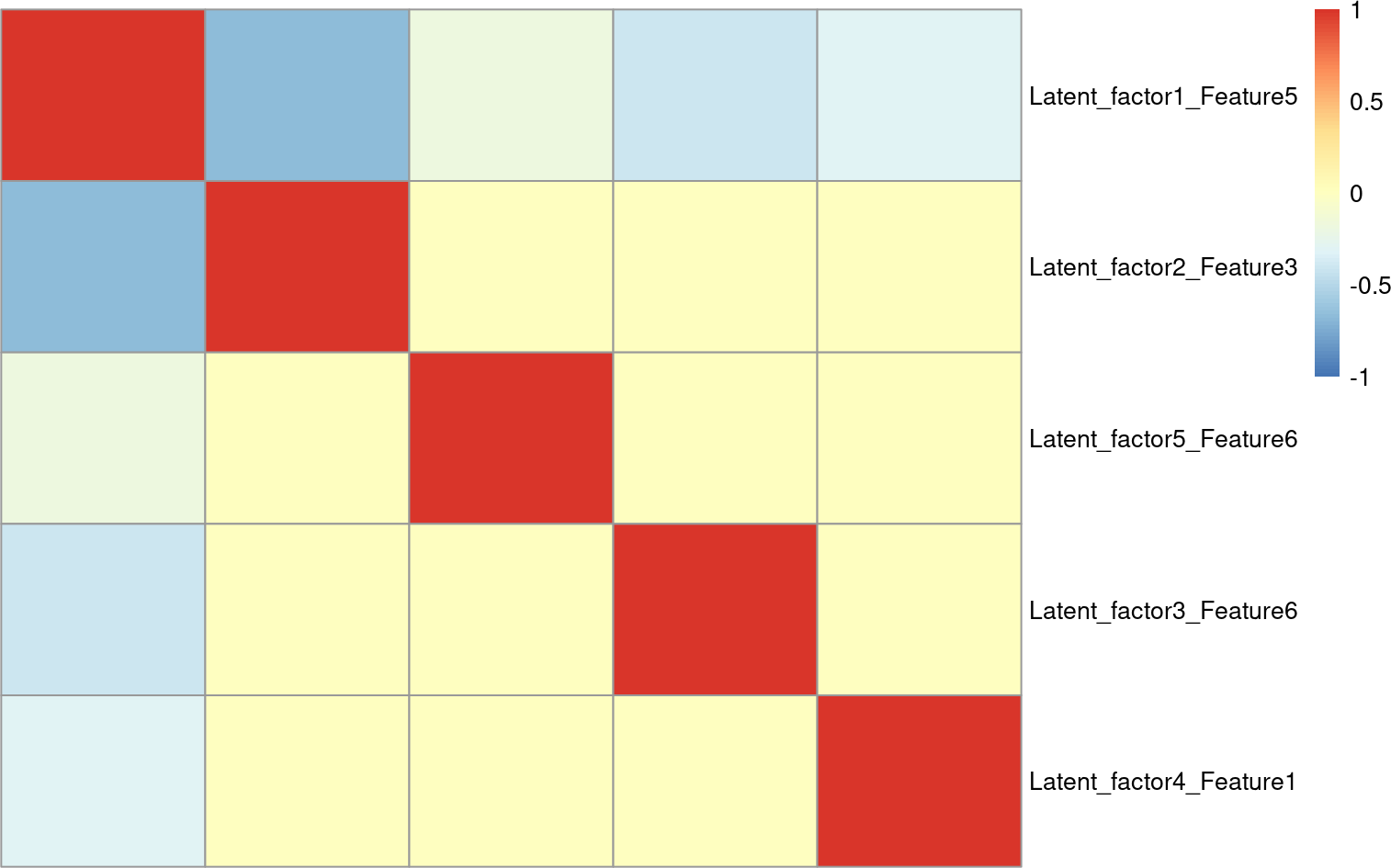``````library(FeatSeekR)
library(DmelSGI)
library(pheatmap)
library(SummarizedExperiment)``````

# 1 Introduction

A fundamental step in many analyses of high-dimensional data is dimension reduction. Feature selection is one approach to dimension reduction whose strengths include interpretability, conceptual simplicity, transferability and modularity. Here, we introduce the `FeatSeekR` algorithm, which selects features based on the consistency of their signal across replicates and their non-redundancy. It takes a 2 dimensional array (features x samples) of replicated measurements and returns a SummarizedExperiment object storing the selected features ranked by reproducibility.

# 2 Installation

``````if (!requireNamespace("BiocManager", quietly = TRUE))
install.packages("BiocManager")

BiocManager::install("FeatSeekR")``````

# 3 Feature selection on simulated data

Here we simulate a data set with features generated by orthogonal latent factors. Features derived from the same latent factor are highly redundant and form distinct clusters. The function simulates 10 redundant features per latent factor. Replicates are generated by adding independent Gaussian noise.

``````set.seed(111)
# simulate data with 500 conditions, 3 replicates and 5 latent factors
conditions <- 500
latent_factors <- 5
replicates <- 3

# simData generates 10 features per latent_factor, so choosing latent_factors=5
# will generate 50 features.
# we simulate samples from 500 independent conditions per replicate. setting
# conditions=500 and replicates=3 will generate 1500 samples, leading to
# final data dimensions of 50 features x 1500 samples
sim <- simData(conditions=conditions, n_latent_factors=latent_factors,
replicates=replicates)

# show that simulated data dimensions are indeed 50 x 1500
dim(assay(sim, "data"))``````
``##    50 1500``
``````# calculate the feature correlation for first replicate
data <- t(assay(sim, "data"))
cor <- cor(data, use = "pairwise.complete.obs")

# plot a heatmap of the features and color features according to their
# generating latent factors
anno <- data.frame(Latent_factor = as.factor(rep(1:5, each=10)))
rownames(anno) <- dimnames(sim)[]
colors        <- c("red", "blue", "darkorange", "darkgreen", "black")
names(colors) <- c("1", "2", "3", "4", "5")
anno_colors <- list(Latent_factor = colors)
range <- max(abs(cor))
pheatmap(cor, treeheight_row = 0 , treeheight_col = 0,
show_rownames = FALSE, show_colnames = FALSE,
breaks = seq(-range, range, length.out = 100), cellwidth = 6,
cellheight = 6, annotation_col = anno, annotation_colors = anno_colors,
fontsize = 8)``````We first plot the correlation matrix of the data to visualize feature redundancy. As intended by the simulation, the features derived from the same latent factor cluster together. This suggests that the true dimension is indeed lower than the number of features. We now run `FeatSeekR` to rank the features based on their uniqueness and reproducibility.

``````# select the top 5 features
res <- FeatSeek(sim, max_features=5)``````
``````## Input data has:
## 1500 samples
## 500 conditions
## 50 features
## 3 replicates``````
``## Starting feature ranking!``
``## Iteration: 1 selected = Latent_factor2_Feature3, replicate F-statistic = 10873.0023316711``
``## Iteration: 2 selected = Latent_factor5_Feature6, replicate F-statistic = 9364.10884704126``
``## Iteration: 3 selected = Latent_factor3_Feature6, replicate F-statistic = 6048.5339756004``
``## Iteration: 4 selected = Latent_factor4_Feature1, replicate F-statistic = 1777.09431418772``
``## Iteration: 5 selected = Latent_factor1_Feature5, replicate F-statistic = 60.3857000568657``
``## Finished feature ranking procedure!``
``## Calculating explained variance of selected feature sets!``
``````# plot a heatmap of the top 5 selected features
plotSelectedFeatures(res)``````We again visualize the selected features by plotting their correlation matrix. As expected, the top 5 selected features are each from a different latent factor and low correlated. This suggests that we were able to obtain a compressed version of the data, while keeping most of the contained information.

# 4 Selecting image features from the `DmelSGI` package

Here we use `FeatSeekR` to rapidly identify unique features with reproducible signal between measurements in an image dataset from the DmelSGI package. The authors of DmelSGI performed combinatorial gene knock-outs using siRNA, followed by imaging of the cells. The resulting images were segmented and features were extracted using the EBImage package. Here, conditions refer to different gene knock-outs, features to the extracted image features and replicates to repeated measurements of the individual conditions.

``````# load data from DmelSGI package
data("subSampleForStabilitySelection", package="DmelSGI")
data <- subSampleForStabilitySelection\$D
# dimensions are conditions, features, replicates
data <- aperm(data, c(1, 3, 2))
# set feature names
dimnames(data)[] <- subSampleForStabilitySelection\$phenotype
# bind samples and create condition factor
conds <- rep(seq_len(dim(data)), 2)
data<- rbind(data[, , 1], data[, , 2])
# show final data dimensions
dim(data)``````
``##  6000  162``

The input data has 3000 samples, 162 features and 2 replicates. Again, we plot the correlation matrix of the data to explore the structure of the features.

``````# calculate correlation matrix of the first 50 features of one of the replicates
cor_mat <- cor(data[, 1:50, drop=FALSE])

# plot correlation matrix, omitting featurenames
pheatmap(cor_mat, show_rownames=FALSE, show_colnames=FALSE,
treeheight_row=0, treeheight_col=0)``````